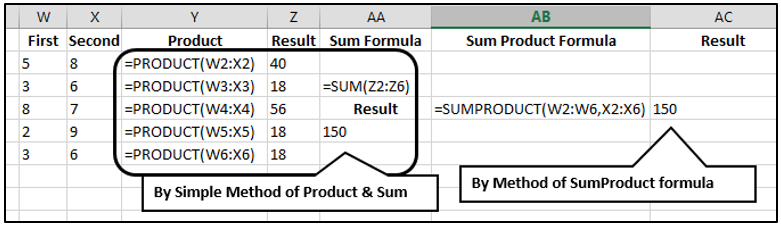Arithmetic Functions in Ms-Excel 2013Arithmetic Functions in Excel 2013

ABS() -  This functions returns an absolute value of the given in the function as an argument. This function display number without any ‘+’ or ‘–’ value.

Syntax-      =abs(Number)

Example-   =abs(-5)

Ans-      5

Sqrt() à This function returns a number after calculate the square root of the given value.

Syntax-     =sqrt(Number)

Example-       =sqrt(16)        Ans-    4

For Negative value-     =sqrt(abs(-16))        Ans-   4

Power() à This function returns a value after calculating the second number is the power of first number.

Syntax-     =power(Number, power)

Example-       =power(10, 2)        Ans-    100

Sum()- this function returns a number after the sum of given number or cell reference as arguments.

Syntax-     =sum(Number1, Number2, Number3, ..., ..)

Example-       =sum(10, 12)        Ans-    22

=sum(c2,d2,e4,f5)

=sum(a1:c5)

=sum(a1:b5,d2:e6)

=sum(a1:b5,10)

=sum(a1:b5,-5)

Sumsq() – This function returns the sum of the squares of the argument as numbers.

Syntax-     =sumsq(Number1, Number2, Number3, ..., ..)

Example-       =sumsq(10, 12)        Ans-    244

=sumsq(c2,d2,e4,f5)

=sumsq(a1:c5)

=sumsq(a1:b5,d2:e6)

=sumsq(a1:b5,10)

Sumproduct() – This function returns the sum of product of corresponding range.

Syntax-     =sumproduct(range1, range2,... ... )

ExampleProduct()- This function returns a number after the multiplication of given number or cell-reference as arguments.

Syntax-     =product(Number1, Number2,... ... )

Example-     =product(5,10,18) à Ans. 9000

=product(c2,d2,e4,f5)

=product(a1:c5)

=product(a1:b5,d2:e6)

=product(a1:b5,10)

=product(a1:b5,-5)

Mod()- This function returns the remainder value after the division of number.
Syntax-      =Mod(Number, Divisor)

Example-        =mod(11,5) à Ans. 1

Quotient() – This function returns the quotient value after the division of given number as argument.

Syntax-    =quotient(Number, Divisor)
Example -     =quotient(11,5) à Ans. 2

Round()- This function returns a rounding value according to given argument.

Syntax-    =Round(Number, Num_digits)

Number à is the number we want to round.

Num_digits à which we want you round off a number.

Ø  If we give num_digits is greater than 0 then number is rounded to normally.

Ø  If we give num_digits is 0 then number is rounded to nearest integer.

Ø  If we give num_digits is less than 0 then number is rounded to left of the decimal point.

a.    =round(5.1678, 2)      Ans. 5.17

b.    =round(5.1678, 0)      Ans. 5.

Example-   =round(5.1678, -1)     Ans. 10
=round(5.1678, 0)      Ans. 5

Roundup()- This function returns a rounding value according to given argument.

Syntax-      =Roundup(Number, Num_digits)

Number à is the number we want to round.

Num_digits à which we want you round off a number.

Ø  If we give num_digits is greater than 0 then number is rounded to normally.

Ø  If we give num_digits is 0 then number is rounded up to nearest integer.

Ø  If we give num_digits is less than 0 then number is rounded up to left of the decimal point

Example-   =roundup(5.1678, -1)     Ans. 10
=roundup(5.1678, 0)      Ans. 5

Rounddown()- This function returns a rounding value according to given argument.

Syntax-      =Rounddown(Number, Num_digits)

Number à is the number we want to round.

Num_digits à which we want you round off a number.

Ø  If we give num_digits is greater than 0 then number is rounded to normally.

Ø  If we give num_digits is 0 then number is rounded down to nearest integer.

Ø  If we give num_digits is less than 0 then number is rounded down to left of the decimal point

Example-   =rounddown(5.1678, -1)     Ans. 0
=rounddown(5.1678, 0)      Ans. 5

Trunc()- this function returns a truncating value according to given condition.
Syntax-      =Trunc(Number, Num_digits)

Number à is the number we want to round.

Num_digits à which we want you round a number. By default it is 0.

Ø  If we give num_digits is greater than 0 then number is rounded to normally.

Ø  If we give num_digits is 0 then number is rounded down to nearest integer.

Ø  If we give num_digits is less than 0 then number is rounded down to left of the decimal point.

a.    =trunc(5.1678, 2)       Ans. 5.16

b.    =trunc(5.1678, 0)       Ans. 5.

c.     =trunc(5.1678, -1)      Ans. 0

Fact() – This function returns a factorial value of the given argument.

Syntax-     Fact(Number)

Example-   =Fact(5)          Ans. 120

Roman() – This function converts decimal number to Roman.
Syntax-    =Roman(Number)
Example-  =roman(10)   Ans. X

Arabic() – This function converts roman number to Arabic number. Given argument of Roman must be as a text format.
Syntax-     =Arabic(Text)

Example-   =Arabic(“X”)   Ans. 10

Int() – This function rounds a number down to nearest integer.

Syntax-     =Int(Real_Num)

Example-     =int(9.7)  Ans. 9

Base() – This function converts a number into other number format according to give its base value and length.

base-      2  for Binary,        8  for Octal,        10   for Decimal,     16   for Hexadecimal

Syntax-       =Base(Num, base, [Min_Len])

Example-     =base(12,2,4)      Ans. 1100 (convert in binary of 12 in 4 digits)

Degrees() – This function is used to converts Radians into degrees.

Syntax-        =Degrees(Angle)

Example-       =degrees(90)   Ans. 5156.62016

LCM() – This function returns the Least Common Multiples of the given numbers as arguments.
Syntax-      =Lcm(Num1, Num2,…)

Example-    ==lcm(2,4,8)  Ans. 8

Log()- this function returns a logarithmic value of the given argument.
Syntax-       =Log(Num1, [base])

Example-        =log(100,10)   Ans. 2

Pi()- This function returns the value of pi.

Syntax-          =pi()

Example-        =pi()   Ans. 3.141592654